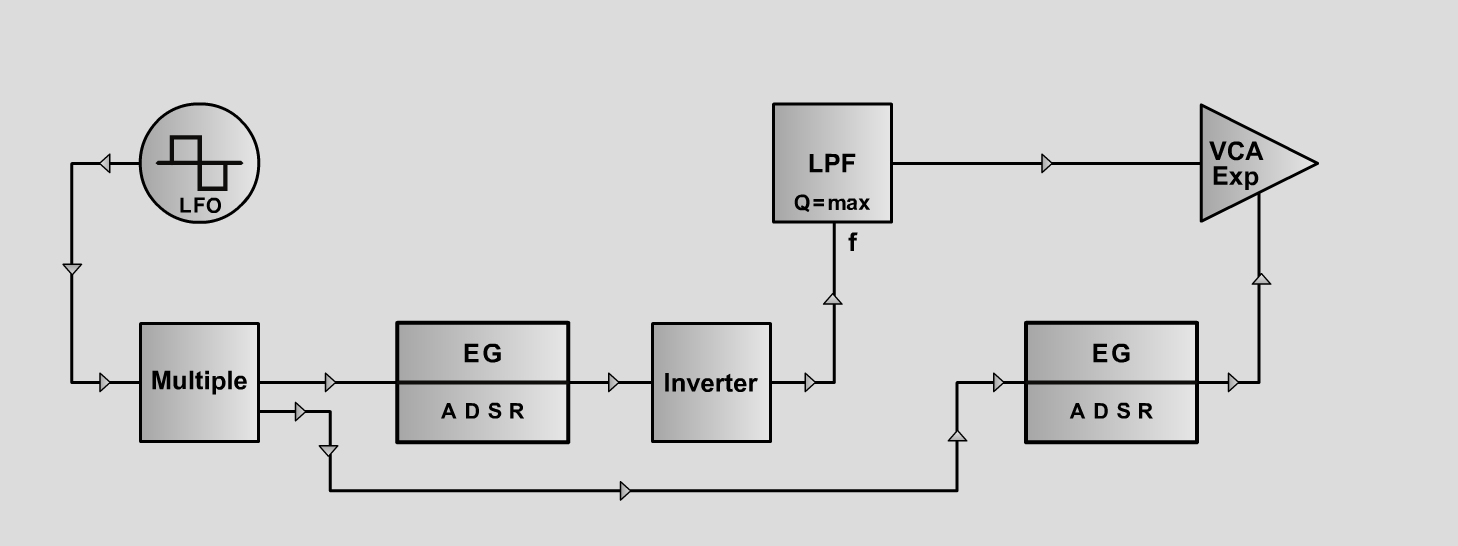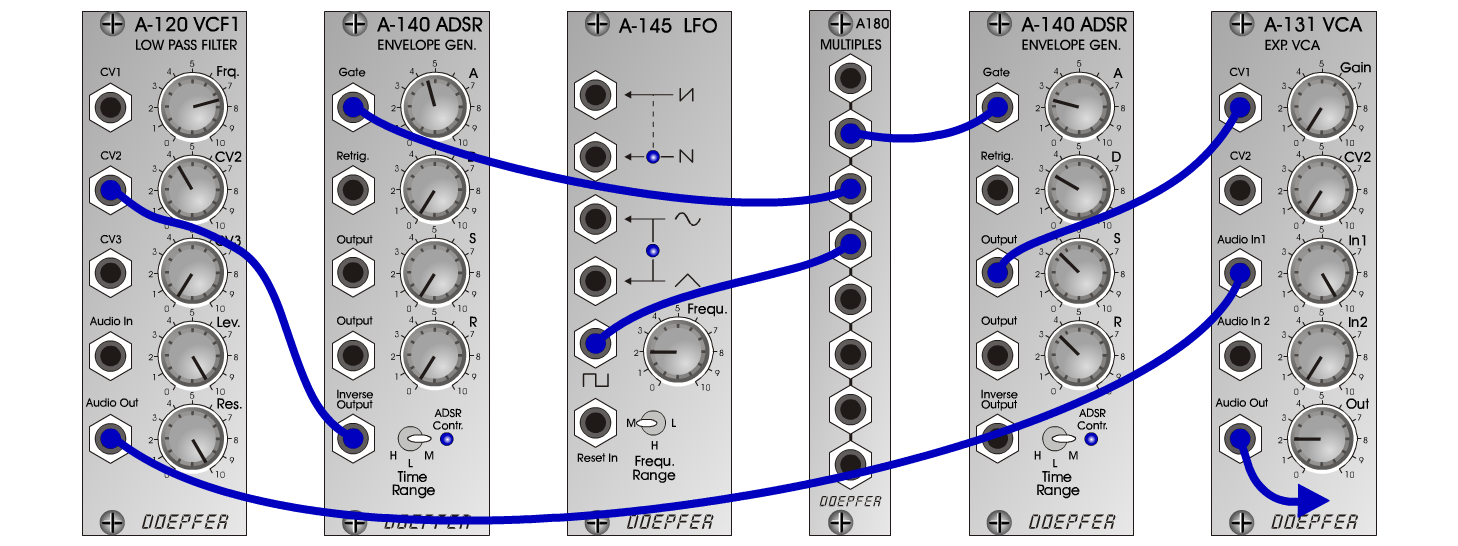Little BirdN a t u r eD o e p f e r A - 100 Connections: Settings: Little Bird 01 A-120 (Audio Out) <=> A-131 (Audio In 1)  A-140/1 (Output) <=> A-131 (CV1)  A-140/2 (Inverse Out) <=> A-120 (CV2)  A-145 (Pulse) <=> A-180  A-180 <=> A-140/1 (Gate)  A-180 <=> A-140/2 (Gate) A-111 (Octave = +1, Tune = 0, Fine = 0, CV 2 = 5) A-120 (Freq = 2.5, Lev = 10, Res = 7.5)  A-131 (Gain = 0, Audio In 1 = 10,  Audio Out = 10)  A-140/1 (A = 0, D = 9, S = 2, R = 10, Range = M)  A-140/2 (A = 0, D = 7.5, S = 8, R = 10, Range = M) Little Bird 02 A-120 (Freq = 7, CV2 = 4, Lev = 10, Res = 10)  A-140/1 (A = 2.5, D = 3, S = 3.5, R = 3.5, Range = M)  A-140/2 (A = 4.5, D = 0, S = 0, R = 0, Range = M)  A-145 (Frq = 2, Range = M)  A-131 (Gain = 0, Audio In 1 = 10, Audio Out = 2) Little Bird 03 A-120 (Freq = 8, CV2 = 4, Lev = 10, Res = 10)  A-140/1 (A = 2.3, D = 3, S = 3.5, R = 3.5, Range = M)  A-140/2 (A = 4.5, D = 0, S = 0, R = 0, Range = M)  A-145 (Frq = 2, Range = M)  A-131 (Gain = 0, Audio In 1 = 10, Audio Out = 2) Little Bird 04 A-120 (Freq = 7, CV2 = 4, Lev = 10, Res = 10)  A-140/1 (A = 2.7, D = 3, S = 3.5, R = 3.5, Range = M)  A-140/2 (A = 6.2, D = 0, S = 0, R = 0, Range = M)  A-145 (Frq = 2, Range = M)  A-131 (Gain = 0, Audio In 1 = 10, Audio Out = 2) Little Bird 05 A-120 (Freq = 6.5, CV2 = 4, Lev = 10, Res = 10)  A-140/1 (A = 2.7, D = 3, S = 3.5, R = 3.5, Range = M)  A-140/2 (A = 6.5, D = 0, S = 0, R = 0, Range = M)  A-145 (Frq = 2, Range = M)  A-131 (Gain = 0, Audio In 1 = 10, Audio Out = 2) Little Bird 06 A-120 (Freq = 7.5, CV2 = 4, Lev = 10, Res = 10)  A-140/1 (A = 2.7, D = 3, S = 3.5, R = 3.5, Range = M)  A-140/2 (A = 6.5, D = 0, S = 0, R = 0, Range = M)  A-145 (Frq = 2, Range = M)  A-131 (Gain = 0, Audio In 1 = 10, Audio Out = 2) Little Bird 07 A-120 (Freq = 8.5, CV2 = 4, Lev = 10, Res = 10)  A-140/1 (A = 2.8, D = 2.3, S = 0, R = 0, Range = M)  A-140/2 (A = 3.1, D = 0, S = 0, R = 0, Range = M)  A-145 (Frq = 2, Range = M)  A-131 (Gain = 0, Audio In 1 = 10, Audio Out = 2) Little Bird 08 A-120 (Freq = 9, CV2 = 4, Lev = 10, Res = 10)  A-140/1 (A = 3, D = 4, S = 0, R = 0, Range = M)  A-140/2 (A = 3, D = 2, S = 0, R = 0, Range = M)  A-145 (Frq = 2, Range = M)  A-131 (Gain = 0, Audio In 1 = 10, Audio Out = 2) Little Bird 09 A-120 (Freq = 8, CV2 = 4, Lev = 10, Res = 10)  A-140/1 (A = 2.7, D = 3.8, S = 0, R = 0, Range = M)  A-140/2 (A = 3, D = 2, S = 0, R = 0, Range = M)  A-145 (Frq = 2, Range = M)  A-131 (Gain = 0, Audio In 1 = 10, Audio Out = 2) Little Bird 10 A-120 (Freq = 5, CV2 = 5, Lev = 10, Res = 10)  A-140/1 (A = 2.7, D = 3, S = 3.5, R = 3.5, Range = M)  A-140/2 (A = 5, D = 0, S = 0, R = 0, Range = M)  A-145 (Frq = 2, Range = M)  A-131 (Gain = 0, Audio In 1 = 10, Audio Out = 2) Little Bird 11 A-120 (Freq = 5, CV2 = 5, Lev = 10, Res = 10)  A-140/1 (A = 2.7, D = 3, S = 3.5, R = 3.5, Range = M)  A-140/2 (A = 6, D = 0, S = 0, R = 0, Range = M)  A-145 (Frq = 2, Range = M)  A-131 (Gain = 0, Audio In 1 = 10, Audio Out = 2) Little Bird 12 A-120 (Freq = 5, CV2 = 5, Lev = 10, Res = 10)  A-140/1 (A = 2.7, D = 3, S = 3.5, R = 3.5, Range = M)  A-140/2 (A = 7, D = 0, S = 0, R = 0, Range = M)  A-145 (Frq = 2, Range = M)  A-131 (Gain = 0, Audio In 1 = 10, Audio Out = 2) Little Bird 13 A-120 (Freq = 5, CV2 = 5, Lev = 10, Res = 10)  A-140/1 (A = 2.7, D = 3, S = 3.5, R = 3.5, Range = M)  A-140/2 (A = 8, D = 0, S = 0, R = 0, Range = M)  A-145 (Frq = 2, Range = M)  A-131 (Gain = 0, Audio In 1 = 10, Audio Out = 2) Notes: Inspired by Isao Tomita�s little bird in Daphnis et Chloe. Josef MuellerSound samples Little Bird Piece.mp3 Little Bird 1 Little Bird 2 Little Bird 3 Little Bird 4 Little Bird 5 Little Bird 6 Little Bird 7 Little Bird 8 Little Bird 9 Little Bird 10 Little Bird 11 Little Bird 12 Little Bird 13